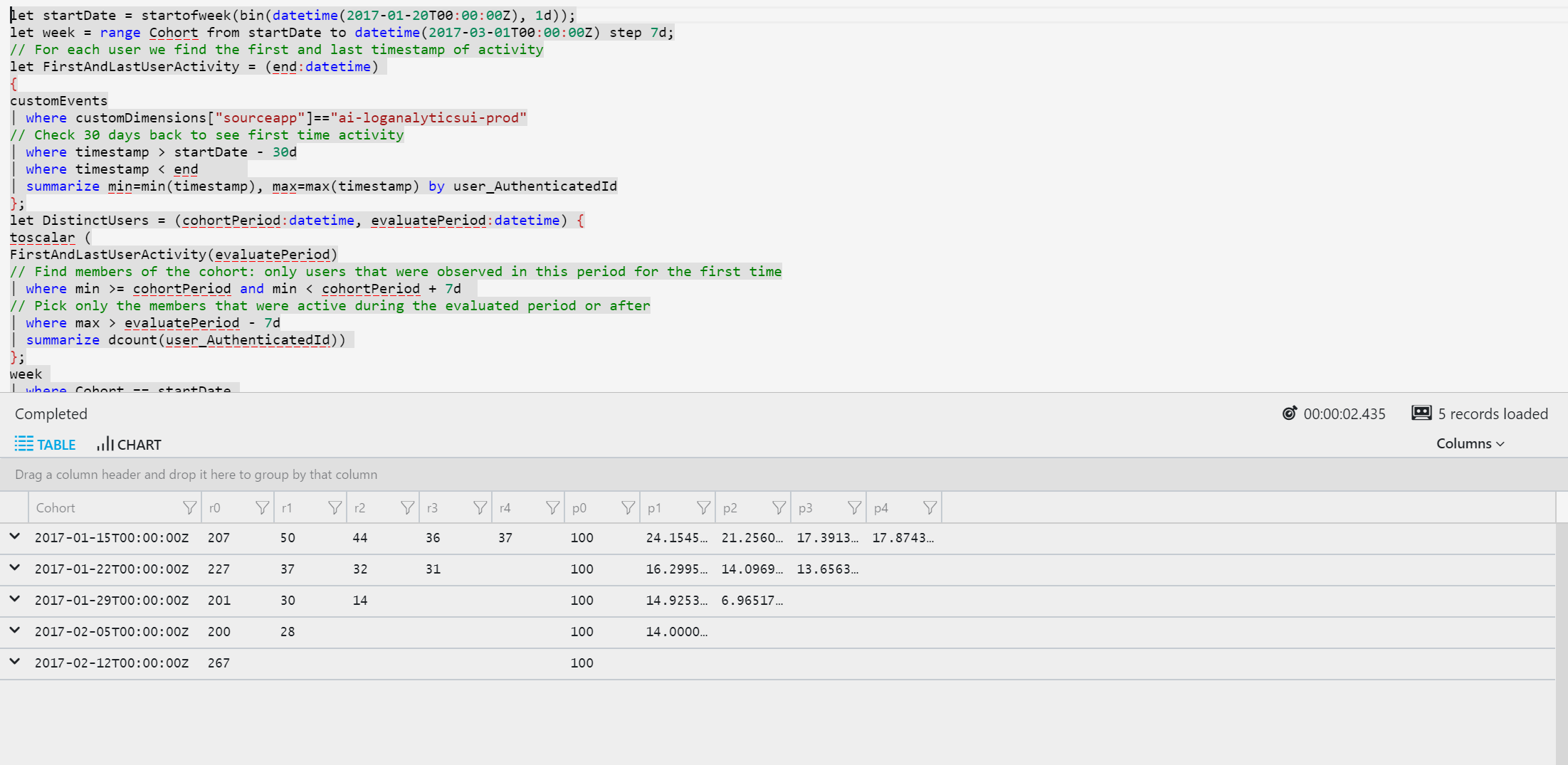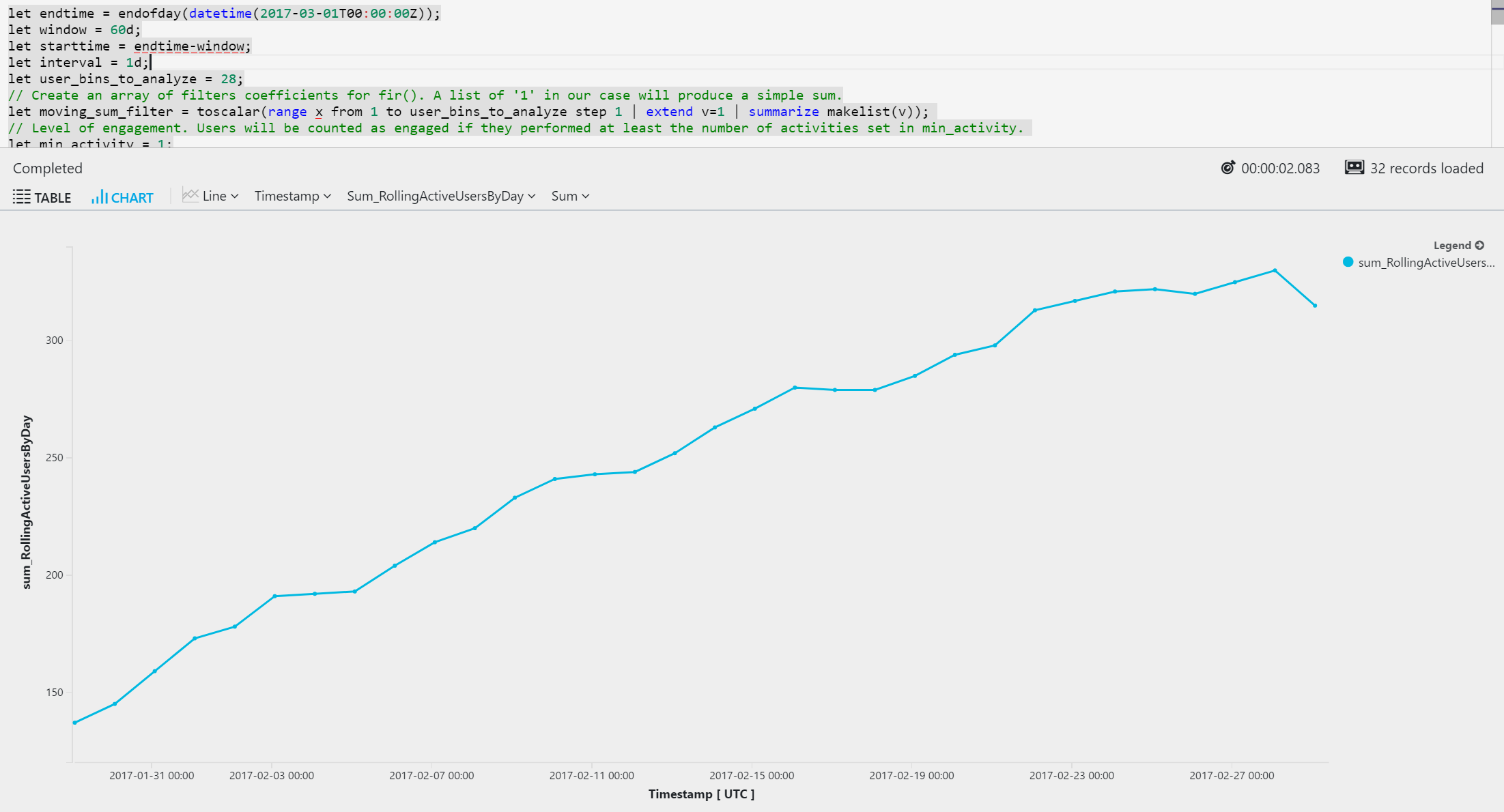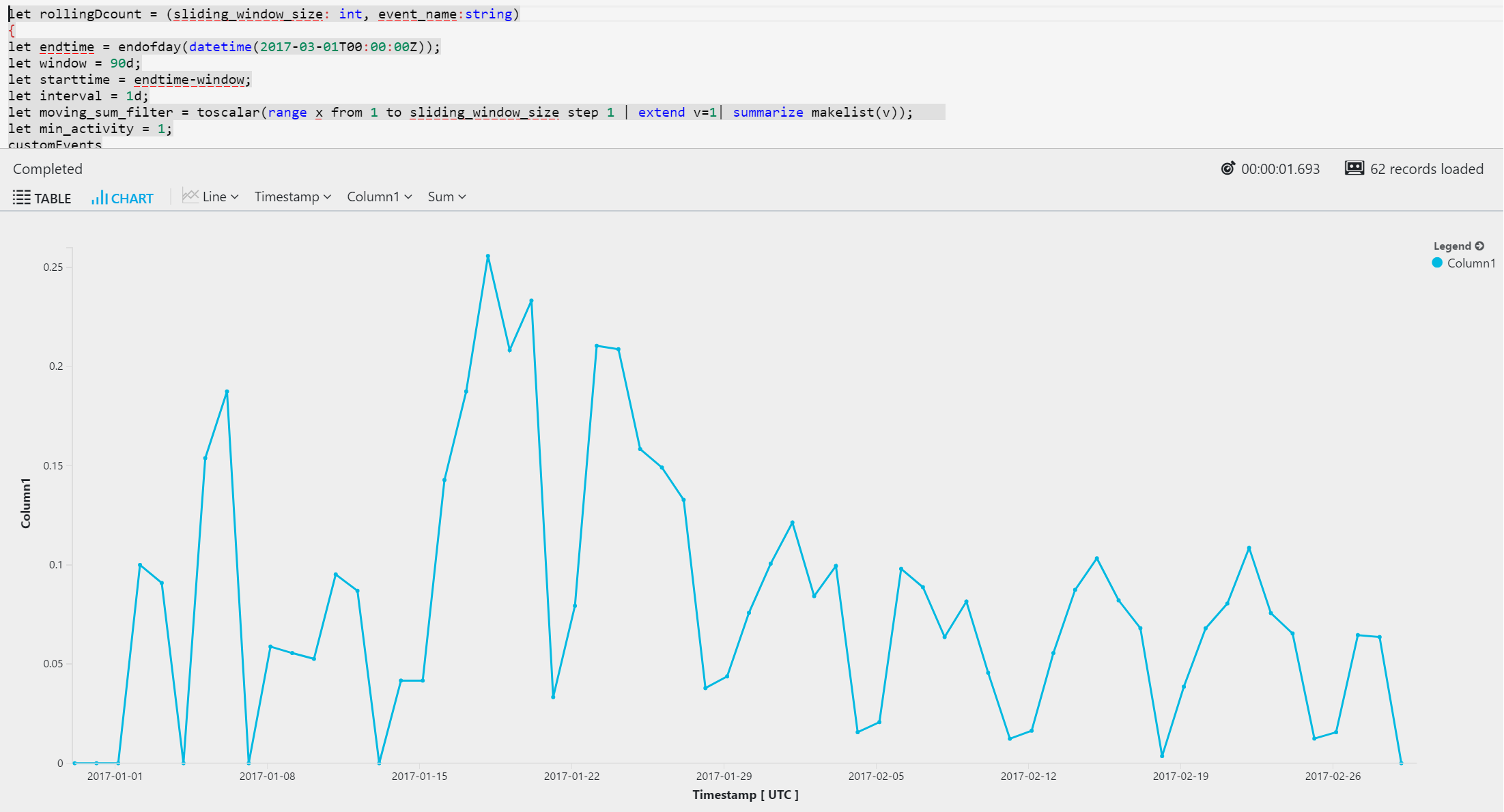# Log Analytics 智能分析示例Log Analytics smart analytics examples

## 队列分析Cohorts analytics

``````let startDate = startofweek(bin(datetime(2017-01-20T00:00:00Z), 1d));
let week = range Cohort from startDate to datetime(2017-03-01T00:00:00Z) step 7d;
// For each user we find the first and last timestamp of activity
let FirstAndLastUserActivity = (end:datetime)
{
customEvents
| where customDimensions["sourceapp"]=="ai-loganalyticsui-prod"
// Check 30 days back to see first time activity
| where timestamp > startDate - 30d
| where timestamp < end
| summarize min=min(timestamp), max=max(timestamp) by user_AuthenticatedId
};
let DistinctUsers = (cohortPeriod:datetime, evaluatePeriod:datetime) {
toscalar (
FirstAndLastUserActivity(evaluatePeriod)
// Find members of the cohort: only users that were observed in this period for the first time
| where min >= cohortPeriod and min < cohortPeriod + 7d
// Pick only the members that were active during the evaluated period or after
| where max > evaluatePeriod - 7d
| summarize dcount(user_AuthenticatedId))
};
week
| where Cohort == startDate
// Finally, calculate the desired metric for each cohort. In this sample we calculate distinct users but you can change
// this to any other metric that would measure the engagement of the cohort members.
| extend
r0 = DistinctUsers(startDate, startDate+7d),
r1 = DistinctUsers(startDate, startDate+14d),
r2 = DistinctUsers(startDate, startDate+21d),
r3 = DistinctUsers(startDate, startDate+28d),
r4 = DistinctUsers(startDate, startDate+35d)
| union (week | where Cohort == startDate + 7d
| extend
r0 = DistinctUsers(startDate+7d, startDate+14d),
r1 = DistinctUsers(startDate+7d, startDate+21d),
r2 = DistinctUsers(startDate+7d, startDate+28d),
r3 = DistinctUsers(startDate+7d, startDate+35d) )
| union (week | where Cohort == startDate + 14d
| extend
r0 = DistinctUsers(startDate+14d, startDate+21d),
r1 = DistinctUsers(startDate+14d, startDate+28d),
r2 = DistinctUsers(startDate+14d, startDate+35d) )
| union (week | where Cohort == startDate + 21d
| extend
r0 = DistinctUsers(startDate+21d, startDate+28d),
r1 = DistinctUsers(startDate+21d, startDate+35d) )
| union (week | where Cohort == startDate + 28d
| extend
r0 = DistinctUsers (startDate+28d, startDate+35d) )
// Calculate the retention percentage for each cohort by weeks
| project Cohort, r0, r1, r2, r3, r4,
p0 = r0/r0*100,
p1 = todouble(r1)/todouble (r0)*100,
p2 = todouble(r2)/todouble(r0)*100,
p3 = todouble(r3)/todouble(r0)*100,
p4 = todouble(r4)/todouble(r0)*100
| sort by Cohort asc
``````## 滚动每月活动用户数和用户粘性Rolling monthly active users and user stickiness

``````let endtime = endofday(datetime(2017-03-01T00:00:00Z));
let window = 60d;
let starttime = endtime-window;
let interval = 1d;
let user_bins_to_analyze = 28;
// Create an array of filters coefficients for series_fir(). A list of '1' in our case will produce a simple sum.
let moving_sum_filter = toscalar(range x from 1 to user_bins_to_analyze step 1 | extend v=1 | summarize makelist(v));
// Level of engagement. Users will be counted as engaged if they performed at least this number of activities.
let min_activity = 1;
customEvents
| where timestamp > starttime
| where customDimensions["sourceapp"] == "ai-loganalyticsui-prod"
// We want to analyze users who actually checked-out in our web site
| where (name == "Checkout") and user_AuthenticatedId <> ""
// Create a series of activities per user
| make-series UserClicks=count() default=0 on timestamp
in range(starttime, endtime-1s, interval) by user_AuthenticatedId
// Create a new column containing a sliding sum.
// Passing 'false' as the last parameter to series_fir() prevents normalization of the calculation by the size of the window.
// For each time bin in the *RollingUserClicks* column, the value is the aggregation of the user activities in the
// 28 days that preceded the bin. For example, if a user was active once on 2016-12-31 and then inactive throughout
// January, then the value will be 1 between 2016-12-31 -> 2017-01-28 and then 0s.
| extend RollingUserClicks=series_fir(UserClicks, moving_sum_filter, false)
// Use the zip() operator to pack the timestamp with the user activities per time bin
| project User_AuthenticatedId=user_AuthenticatedId , RollingUserClicksByDay=zip(timestamp, RollingUserClicks)
// Transpose the table and create a separate row for each combination of user and time bin (1 day)
| mvexpand RollingUserClicksByDay
| extend Timestamp=todatetime(RollingUserClicksByDay)
// Mark the users that qualify according to min_activity
| extend RollingActiveUsersByDay=iff(toint(RollingUserClicksByDay) >= min_activity, 1, 0)
// And finally, count the number of users per time bin.
| summarize sum(RollingActiveUsersByDay) by Timestamp
// First 28 days contain partial data, so we filter them out.
| where Timestamp > starttime + 28d
// render as timechart
| render timechart
````````````let rollingDcount = (sliding_window_size: int, event_name:string)
{
let endtime = endofday(datetime(2017-03-01T00:00:00Z));
let window = 90d;
let starttime = endtime-window;
let interval = 1d;
let moving_sum_filter = toscalar(range x from 1 to sliding_window_size step 1 | extend v=1| summarize makelist(v));
let min_activity = 1;
customEvents
| where timestamp > starttime
| where customDimensions["sourceapp"]=="ai-loganalyticsui-prod"
| where (name == event_name)
| where user_AuthenticatedId <> ""
| make-series UserClicks=count() default=0 on timestamp
in range(starttime, endtime-1s, interval) by user_AuthenticatedId
| extend RollingUserClicks=fir(UserClicks, moving_sum_filter, false)
| project User_AuthenticatedId=user_AuthenticatedId , RollingUserClicksByDay=zip(timestamp, RollingUserClicks)
| mvexpand RollingUserClicksByDay
| extend Timestamp=todatetime(RollingUserClicksByDay)
| extend RollingActiveUsersByDay=iff(toint(RollingUserClicksByDay) >= min_activity, 1, 0)
| summarize sum(RollingActiveUsersByDay) by Timestamp
| where Timestamp > starttime + 28d
};
// Use the moving_sum_filter with bin size of 28 to count MAU.
rollingDcount(28, "Checkout")
| join
(
// Use the moving_sum_filter with bin size of 1 to count DAU.
rollingDcount(1, "Checkout")
)
on Timestamp
| project sum_RollingActiveUsersByDay1 *1.0 / sum_RollingActiveUsersByDay, Timestamp
| render timechart
``````## 回归分析Regression analysis

• 使用 make-series 将半结构化文本跟踪日志转换为指标，用于表示正跟踪线与负跟踪线之间的比率。Use make-series to convert semi-structured textual trace logs into a metric that represents the ratio between positive and negative trace lines.
• 结合时序分析和双线线性回归，使用 series_fit_2linesseries_fit_line 执行高级跳步检测。Use series_fit_2lines and series_fit_line to perform advanced step-jump detection using time-series analysis with a 2-line linear regression.
``````let startDate = startofday(datetime("2017-02-01"));
let endDate = startofday(datetime("2017-02-07"));
let minRsquare = 0.8;  // Tune the sensitivity of the detection sensor. Values close to 1 indicate very low sensitivity.
// Count all Good (Verbose + Info) and Bad (Error + Fatal + Warning) traces, per day
traces
| where timestamp > startDate and timestamp < endDate
| summarize
Verbose = countif(severityLevel == 0),
Info = countif(severityLevel == 1),
Warning = countif(severityLevel == 2),
Error = countif(severityLevel == 3),
Fatal = countif(severityLevel == 4) by bin(timestamp, 1d)
| extend Bad = (Error + Fatal + Warning), Good = (Verbose + Info)
// Determine the ratio of bad traces, from the total